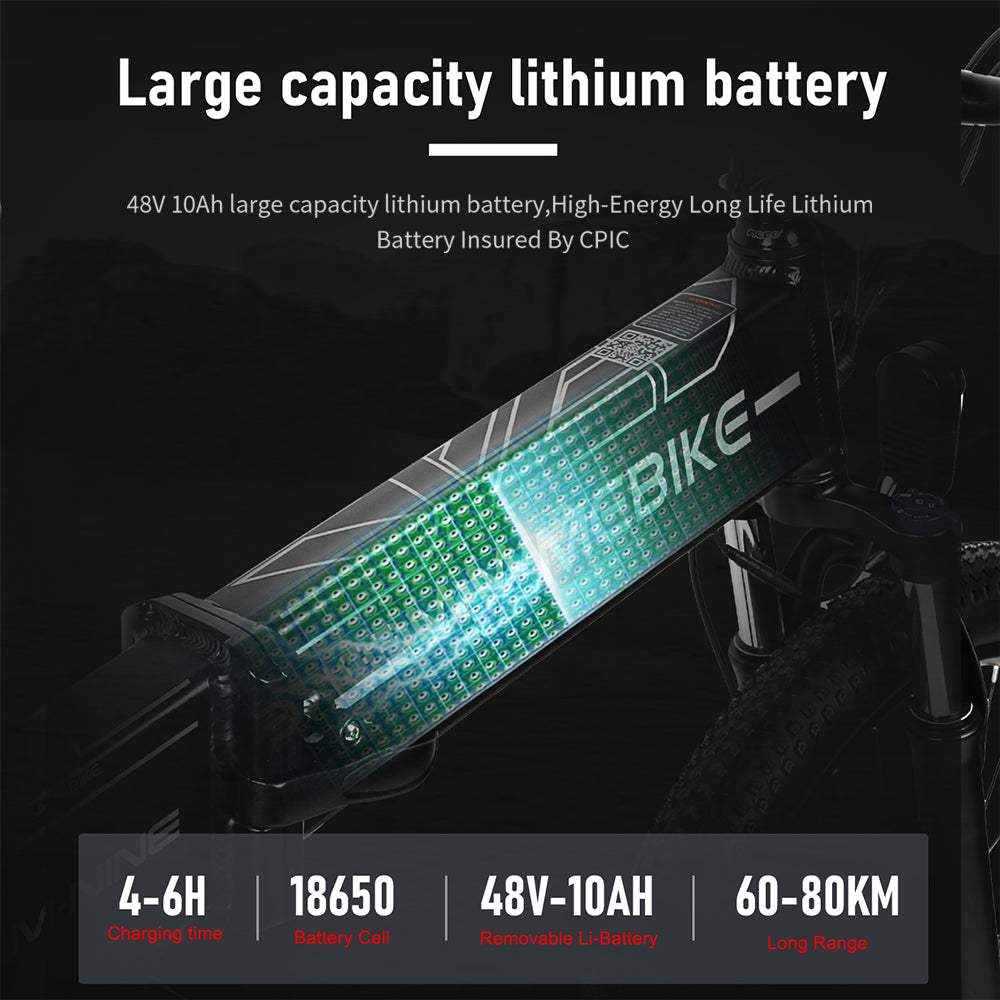Ebike Range: How Long Range Can Your Electric Bike Go?

The longest range of electric bicycle has always been one of the most important issues for riders. After all, no one wants to be embarrassed by running out of power halfway. The ebike range can comprehensively calculate the electric bicycle distance based on the load, battery voltage, battery capacity, battery weight, discharge time, discharge current, etc., so as to konw how long range can your ebike go.The average riding current of different electric bike is different, the continuous discharge time of the battery is different, and the continuation mileage of the electric bike is different, because the efficiency of the motor is the highest when working at rated power, so without considering other external factors When the maximum speed of the ebike is maintained and the ebike is accelerated uniformly, the electric bicycle has the longest riding range. Below are several calculation formulas.

Formula 1: V=P/(G×0.09) (V: fastest speed P: rated power G: total load weight)
Formula 2: I=P/U (P: rated power U: battery voltage I: current)
Formula 3: T=Q/I (Q: battery capacity I: riding current)
Formula 4: S=VT (S: mileage V: vehicle speed T: riding time)

## Battery capacity

Battery capacity refers to the amount of electricity stored in the battery. The battery capacities we usually see are generally 8Ah, 10.5Ah, 12Ah, 13Ah, 14Ah, 17Ah, etc. Different battery capacities are also different. Generally speaking, the larger the battery capacity, the more The larger the battery, the longer the cruising range of the electric bike will be. Of course, the price of the battery will be higher.

## Motor Power

Motor power refers to the power of the motor used in electric bicycle. The power of the motor can affect the speed of the electric bike. Generally speaking, the greater the power of the motor, the faster the electric bike will be. However, the greater the power of the motor, the higher the power consumption, and the shorter the optimal mileage will be. Therefore, motor power has a great impact on electric bicycles. Generally speaking, electric bicycles travel at medium speeds and have the longest range. That is to say, electric bike motors should not run at full load. They must find a middle balance point to ensure that The speed will also reduce the power consumption of the motor.## Voltage

There is a diverse array of battery voltage options available for electric bicycles, each with its own unique characteristics and capabilities. However, it's worth noting that a prevalent and widely adopted voltage rating for electric bicycles is 48 volts. This voltage rating is typically determined by the number of individual batteries utilized in the electric bicycle's power source. Specifically, if each battery contributes 12 volts to the system, then a standard electric bicycle configuration typically employs four batteries in series to achieve a total voltage of 48 volts.

## Speed

Different types of ebike have different speeds. Taking the samebike electric bicycle as an example, the speed of electric commuter bicycles is 32~40km/h, and the speed of off-road electric bicycles and electric mopeds is 40~50km/h. The speed also affects the cruising range of the e-bike. If the speed is too fast, the motor will always output at maximum power, generate a lot of heat, consume more electric energy, and also affect the service life of the motor; if the speed is too slow, frequent braking and restarting will also consume more electric energy, so it is generally believed that Electric bicycles save energy when traveling at medium speeds.

Above we have explained the ebike range calculation formula and various parameters respectively. In order to allow more netizens to understand how to use the calculation formula, we have used two common electric bicycles to calculate their real endurance for everyone mileage.

The first model is the SAMEBIK RS-A01 electric commuter bicycle. It is equipped with a 48V/10.4AH battery. The weight of the bicycle (including battery) is 31KG. Plus the maximum weight of the rider is 110kg, the total load weight is 141kg. The electric bicycle uses With a 350W motor, according to the calculation formula above, we can know that the pure electric cruising range of this electric bicycle at the maximum speed is 55KM.

The second model is the SAMEBIKE M20 electric motorcycle. It is equipped with a 48V/18AH battery. The weight of the bicycle (including battery) is 58KG. With the maximum weight of the rider being 110kg, the total load weight is 168kg. The electric bicycle uses a 1000W motor. , according to the above calculation formula, we can know that the pure electric cruising range of this electric bicycle at the maximum speed is 60KM

The above calculation formula is the theoretical cruising range of an electric bike. The actual cruising range is affected by various factors such as ambient temperature and road conditions, and is shorter than the theoretical cruising range. However, the actual cruising range will not be greater than the theoretical cruising range. Therefore, when we purchase an ebike, we can easily calculate the actual cruising range of the electric bike based on this formula.

### Shop ebikes

Vélo électrique M20 1000W